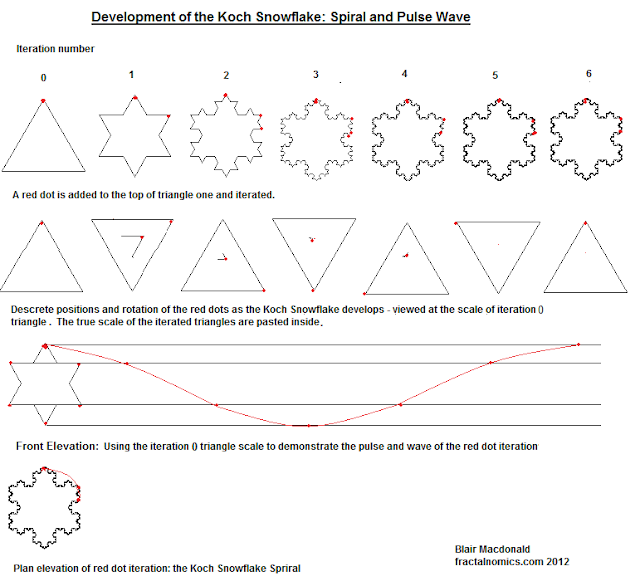### Measuring the fractal wavelength

An attempt to measure the fractal wavelength.
Rational
This entry came (to me) from working on the fractal spiral, the fractal wave, and de Broglie demand curve entries. I thought - as it is impossible to measure both time and distance - that it may be possible to measure - even if only in principle - the distance of the wavelength - based on a known dimension, the length of the triangle side (l).

Background.
Fig. 1 below shows the fractal and its (while developing) 'wave like' nature. It shows the wave takes 6 iterations to repeat or produce one cycle, but it does not show the length of the wave, the wavelength (λ).Fig. 1

Measuring the wavelength, an experiment.
I came to me that through a simple 'experiment', the wavelength (λ) may be measure, or at least better understood.
Method:

1. Take the iteration 0 triangle, and post it on a wall.
2. Cut out from a paper print the iteration 1 triangle from the fully developed Koch snowflake
3. Hold the paper at arms length and sight the triangle 0 through the triangle 1 hole.
4. The arm length will be known as a standard observation distance*
5. Move forward or back with the paper extended at standard distance, and find the place where the triangle 0 appears the same size as triangle 1.
6. Make a mark of this distance.
7. Repeat the process, this time for triangle 2, mark the distance.
8. Repeat the process, this time for triangle 3, mark the distance.
9. Record the distances (in metres) then divide these distances by the triangle base length.
*There is an issue with the 'standard distance': The accuracy and thus validity of the distances measured in process 6,7,8 and 9 are dependent on the standard distance, and as this distance is subjective, there is a measurement problem. And thus no standard distance.
More to come.